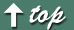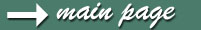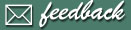## How to measure speaker impedance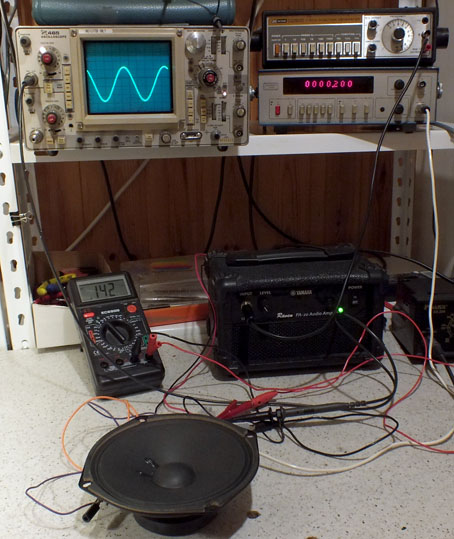If you try to measure speaker impedance using ordinary resistance meter, or DVM, you don't get a correct result. I wanted to learn how to do the measurement properly. It was easy to find instructions from internet. Preferable method is to use a computer with soundcard and suitable software. I wanted to make it manually, old school  way, as a learning experience.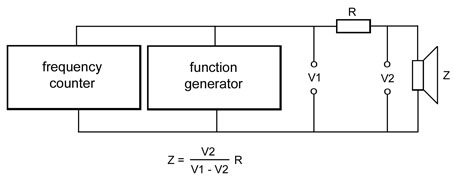This is the system I used. There is also an amplifier after the sine wave generator (I forgot to draw it!) that feeds the signal to the speaker through a series resistor (100 ohms/2W). The idea is to measure ac voltages V1 and V2 at different frequencies. Impedance can then be calculated using the formula above. I wasn't sure how well my old and tired DVM could measure low ac voltages so I used also an oscilloscope. Results show that an ordinary DVM has a limited frequency range, but it was actually quite ok at the area of interest.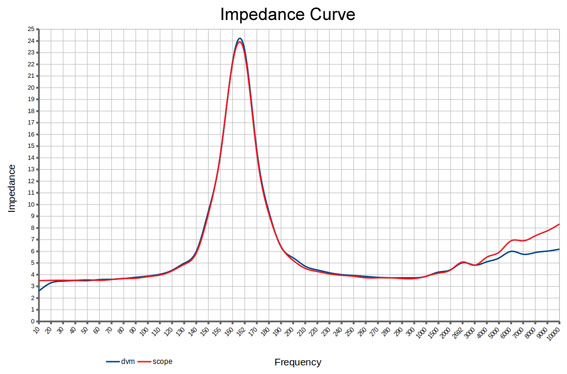I used a spreadsheet to calculate impedance values and to plot the impedance curve. What we want to know is the minimum impedance value above speaker's  fundamental resonance frequency (high peak in the curve). The answer is about 3,7 ohms at 290 Hz, Z(min). Now we can calculate the speaker's rated impedance, as Z(min) / 0,8 =  ~4.6 ohms. Rated impedance is defined so that the minimum impedance may not  be less than 80% of the rated (nominal) impedance. Rated impedance is the value marked on the speaker by manufacturer. So the minimum actual impedance of a 8 ohm speaker can be 0,8 x 8 = 6,4 ohms.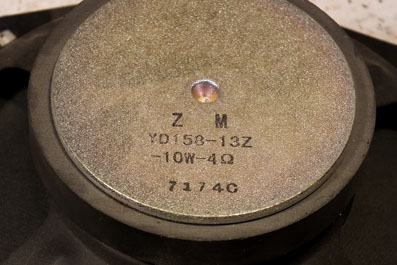For this test I used some unnamed 6 inch speaker I had lying around. My result, 4,6 ohms, is little higher than what is stamped on the speaker. When I measured the dc resistance directly with a DVM I got just 3,3 ohms.